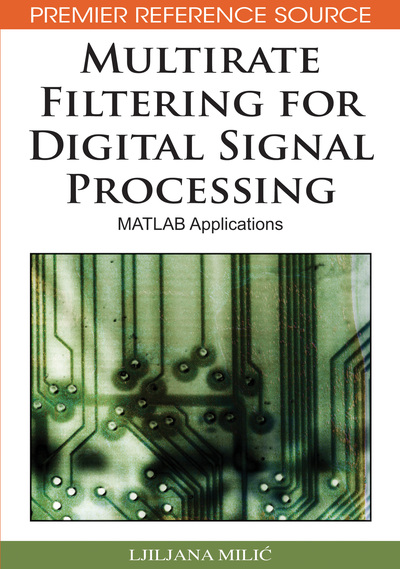# IIR Filters to Sampling Rate Conversion

Ljiljana Milic (Mihajlo Pupin Institute, Serbia)
DOI: 10.4018/978-1-60566-178-0.ch005
Available
\$33.75
List Price: \$37.50
10% Discount:-\$3.75
TOTAL SAVINGS: \$3.75

## Abstract

Infinite impulse response (IIR) filters are used in applications where the computational efficiency is the highest priority. It is well known that an IIR filter transfer function is of a considerably lower order than the transfer function of an FIR equivalent. The drawbacks of an IIR filter are the nonlinear phase characteristic and sensitivity to quantization errors. In multirate applications, the computational requirements for FIR filters can be reduced by the sampling rate conversion factor as demonstrated in Chapter IV. However, such a degree of computation savings cannot be achieved in multirate implementations of IIR filters. This is due to the fact that every sample value computed in the recursive loop is needed for evaluating an output sample. Based on the polyphase decomposition, several techniques have been developed which improve the efficiency of IIR decimators and interpolators as will be shown later on in this chapter. In this chapter, we consider first the direct implementation structures for IIR decimators and interpolators. In the sequel, we demonstrate the computational requirements for direct form IIR decimators and interpolators. The polyphase decomposition of an IIR transfer function is explained with its application to decimation and interpolation. Then, we demonstrate an efficient IIR polyphase structure based on all-pass subfilters, which is applicable to a restricted class of decimators and interpolators. In this chapter, we discuss the application of the elliptic minimal Q factor (EMQF) filter transfer function in constructing high-performance decimators and interpolators. The chapter concludes with a selection of MATLAB exercises for the individual study.
Chapter Preview
Top

## Direct Implementation Structures For Iir Filters For Decimation And Interpolation

For studying the efficiency of IIR decimators and interpolators let us consider the factor-of-M decimator depicted in Figure 1, where the decimation filter H(z) is an Nth order IIR transfer function.

For an IIR decimation filter, the H(z) is the transfer function of the form, (1) where A(z) and B(z) are Nth order polynomials in z-1,. (2)

The input-output relations (1) can be rearranged in a product of the recursive section 1/A(z) and nonrecursive section B(z),. (3)

Denoting the output of the recursive part with W(z), i.e.,

(4) we present relation (3) as the output of the nonrecursive part inputted by W(z),. (5)

In time domain, input-output relations (4) and (5) are expressed by the difference equations,, (6). (7)

The recursive equation (6) computes the intermediate sequence {w[n]}, which is used in the nonrecursive equation (7) for computing the output samples v[n].

## Complete Chapter List

Search this Book:
Reset